# Study Guide

## MoGEA Mathematics SubtestSample Questions

### Competency 0006Understand numbers and algebra.

1. At a community plant sale, a gardener buys 60 plants. Some cost \$5 per plant and the rest cost \$3 per plant. The total cost of the plants is \$236. If x represents the number of \$3 plants, which of the following equations could be solved to find the number of \$3 plants purchased?

1. 3x + 5(60 - x) – 236 = 0
2. 5x + 3(60 – x) – 236 = 0
3. 3x + 5(x – 60) – 236 = 0
4. 5x + 3(x – 60) – 236 = 0
Correct Response: A. This question requires the examinee to represent and solve real-world problems using algebraic expressions and equations. If the gardener purchased x plants that cost \$3 each and 60 plants altogether, then the gardener purchased 60 – x plants that cost \$5 each. The value of the \$3 plants is 3x and the value of the \$5 plants is 5(60 – x). As the total value of the purchase was \$236, the equation that could be solved to find the number of \$3 plants purchased is 3x + 5(60 – x) = 236, or 3x + 5(60 – x) – 236 = 0.

### Competency 0007Understand measurement and geometry.

2. Use the incomplete geometric proof below to answer the question that follows.

 Given: ABCD is a parallelogramAW ≅ XC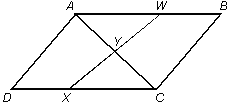Prove: WY ≅ YX Statements ABCD is a parallelogram. AW ≅ XC AB || DC ∠WAC ≅ ∠XCA∠AWX ≅ ∠CXW ΔAWY ≅ ΔCXY WY ≅ YX Reasons Given Given Definition of a parallelogram If parallel lines are crossed by a transversal, alternate interior angles are congruent. ? Corresponding parts of congruent triangles are congruent.

Which of the following is the reason for Statement 5 in the proof above?

1. AAS
2. AAA
3. ASA
4. SAS
Correct Response: C. This question requires the examinee to analyze formal and informal geometric proofs. Side AW is between ∠WAC and ∠AWX, and side XC is between ∠XCA and ∠CXW. It is given that AWXC. Since it has been proven that ∠WAC ≅ ∠XCA and ∠AWX ≅ ∠CXW, the Angle Side Angle (ASA) postulate of triangle congruence is the reason for Statement 5 in the proof.

### Competency 0008Understand probability and statistics.

3. A car rental agency has 30 cars on its lot. Half of the cars are red and half of the cars are blue. A customer rents a red car and drives it away. If the next customer selects a car at random, what is the probability that the car will be red?

1.2.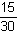3.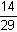4.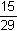Correct Response: C. This question requires the examinee to apply knowledge of the rules of probability and counting to compute probabilities and solve problems. Since half of the 30 cars are red and half are blue, there must be 15 red cars and 15 blue cars on the lot. If a customer rents a red car, there are 14 red cars and 15 blue cars left on the lot. The probability that the next customer will select a red car is the number of red cars (14) over the total number of cars (29), or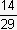.